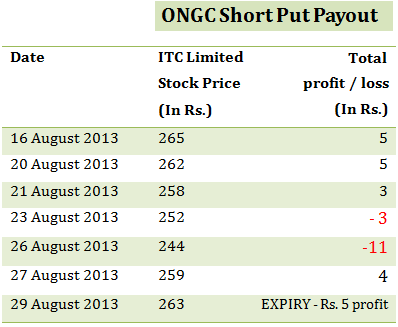When to use: When you are Bullish and anticipate the stock / index to fall.

How it works: Suppose, you are Bullish onon 16th August 2013, when the stock trades at Rs. 265. You sell a put option (also called writing a put option) for a premium of Rs. 5, expiring 29th August 2013 with a Strike Price of Rs. 260.

Risk / Reward: If the price of ONGC stock stays above Rs. 260 (i.e. the strike price) until expiry, you will retain the entire premium amount of Rs. 5. If however the price falls below Rs. 260, the buyer of put option may exercise his option. Once the price falls below Rs. 255 (i.e. the strike price – premium), the buyer of put option will start making a profit based on how far below Rs. 255 does the stock price fall.

From the perspective of the seller of the put (you), you will start suffering a loss once the stock price falls below Rs. 255 (i.e. the strike price – premium).

When you short (or write) an option contract your risk is practically unlimited, as the stock may fall to any level (all the way up to zero) while your reward is limited to the amount of premium (i.e. Rs. 5 in the example) which is the maximum profit which you stand to gain from this trade.

The Chart illustrates your payout on given dates and prices (the table below assumes a scenario where the option gets exercised before expiry. Note that in the first two scenarios the option cannot be exercised as the price of ONGC is above the strike price):As you can see above, the option buyer will not exercise his option so long as the price stays above Rs. 260 (i.e. the strike price). Once the price falls below Rs. 260, the option buyer may exercise his option and you (i.e. seller of put option) may lose part of the premium you earned depending on how far below Rs. 260, the price falls when the option gets exercised. If the option is exercised after the price falls below Rs. 255, you will start making a loss. If you hold on to your position until expiry, it will be settled based on the difference between the closing price of ONGC share and the strike price.

Note: The example and calculations are based assuming a single share though in reality options are based on lots of many shares. For example ONGC Put option contract is for 1000 shares. Accordingly the premium you receive will be Rs. 5,000 for a single lot (i.e. 5 *1000) in our example.

Also Note: Unlike the buyer of an option who only pays the premium to buy the option, the seller of an option must deposit a margin amount with the exchange. This is because he practically takes an unlimited risk as the stock price may fall to any level (up to zero). In case the price falls sharply below the strike price, the exchange utilises the margin amount to make good the profit which the option buyer makes. The amount of margin is decided by the exchange and it typically ranges from 15 % 60 % based on the volatility in the underlying stock and market conditions. In the above example, as a seller of put option, you will have to deposit a margin of Rs. 50,778 (i.e. Strike price * Lot size * 19.53%) for selling/writing a single lot of ONGC put option. Note that the total value of your outstanding position in this case will be Rs. 2,60,000 (i.e. strike price * lot size).